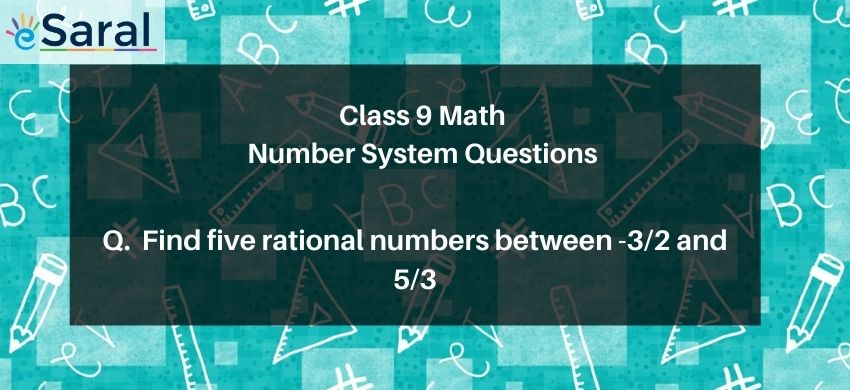Most Affordable JEE | NEET | 8,9,10 Preparation by Kota's Top IITian Doctor Faculties

# Find any five rational numbers between -3/2 and 5/3`

# Question : State true or false :Five rational numbers between $\frac{-3}{2}$ and $\frac{5}{3}$ are $\frac{-8}{6}, \frac{-7}{6}, 0, \frac{1}{6}, \frac{2}{6}$

1. True
2. False
Solution :

The Correct option is 1. True

To get the rational numbers between $\frac{-3}{2}$ and $\frac{5}{3}$

Take an LCM of these two numbers: $\frac{-9}{6}$ and $\frac{10}{6}$

All the numbers between $\frac{-9}{6}$ and $\frac{10}{6}$ form the answer

Some of these numbers are $\frac{-8}{6}, \frac{-7}{6}, 0, \frac{1}{6}, \frac{2}{6}$

Hence the statement is true.